• harris角点检测python实现
2022-06-22 02:25:22
import cv2 as cv
import numpy as np

print(imgo.shape)
imgc = cv.cvtColor(imgo, cv.COLOR_BGR2GRAY)
xbian = imgc.shape
ybian = imgc.shape
def cv_show(img,imgc):
cv.imshow(img,imgc)
cv.waitKey(0)
cv.destroyAllWindows()
cv_show('img',imgc)
sobelx = abs(cv.Sobel(imgc,cv.CV_64F,1,0,ksize=3))#默认ksize=3
sobely = abs(cv.Sobel(imgc,cv.CV_64F,0,1))
for i in range(1,xbian-1):
for j in range(1,ybian-1):
IXX = sobelx[i][j]*sobelx[i][j]
IXY = sobelx[i][j]*sobely[i][j]
IYY = sobely[i][j]*sobely[i][j]
M = np.mat([[IXX,IXY],[IXY,IXX]])
for xx in range(i-1, i+2):
for yy in range(j-1, j+2):
N = np.mat([[abs(xx-i)],[abs(yy-j)]])
E = np.dot(N.T,M)
Z = np.dot(E,N)
aver = []
aver.append(Z)
if np.mean(aver)>3000:
imgo[i][j] = (0,255,0)
cv_show("imgo",imgo)


Harris角点检测
比较潦草，实验课作业，参数可更改。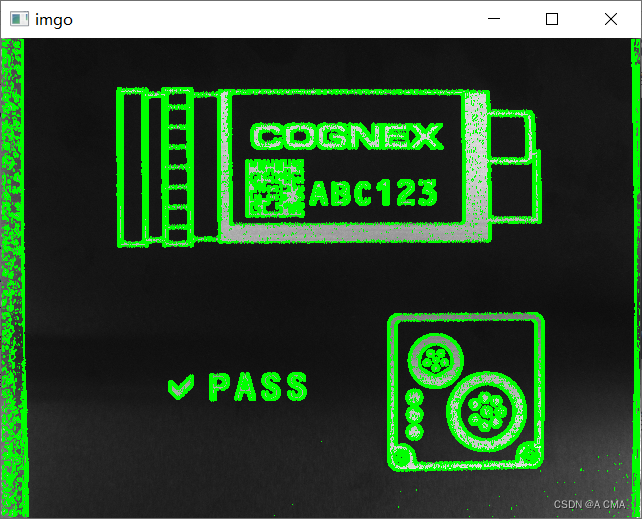python 图像处理 计算机视觉
更多相关内容
• harris角点检测python实现，基于opencv实现，非极大值抑制
• Harris角点检测python实现及基于opencv实现，（角检测、非极大值抑制）

# 写在前面：

七月，骄阳似火， 好想dying in the sun。黄宁然，为何还在这里。

# 参考文献镇楼：

袁志聪，基于harris特征的点云配准方法研究
高亭，基于改进Harris角点检测的印刷体文档图像检索技术
景庆阳，基于harris角点检测的KCF算法研究.
zoukankan，OpenCV计算机视觉学习（13）——图像特征点检测（Harris角点检测，sift算法），
 Harris角点检测原理【OpenCV教程】
 勿在浮砂筑高台，【特征匹配】Harris及Shi-Tomasi原理及源码解析
注：csdn发文助手提示“外链过多”，故文献链接网址请见评论区。

笔者的执念。

# 1、原理简介

Harris算法是chris harris等提出的一种基于信号的点特征提取算法，是对Moravec算子的改良和优化。Moravec算子主要是研究图像的固定窗口在四个不同方向上的移动，比较窗口中的像素灰度变化情况的大小，而harris角点检测则是比较窗口在任意方向滑动前后的灰度变化大小的情况，并且用数学表达式确定特征点的位置；同时引入了平滑因子，进一步增强了抗干扰能力。若窗口移动前后，灰度发生了较大变化，则判断窗口中存在角点，否则窗口内不存在角点。根据文献，具体公式推导如下。
当窗口的偏移量为[j,q]时，滑动前后窗口的灰度变化为：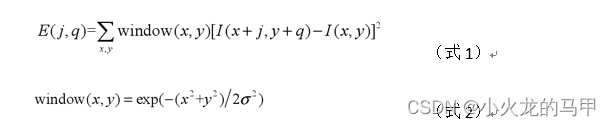式中，Window(x,y)是用来滤波的高斯函数，I(x+j,y+q)为移动后的图像灰度，I(x,y)为原始图像灰度。
根据泰勒公式，二次展开为：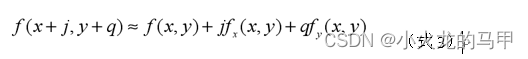则式（1）变换为：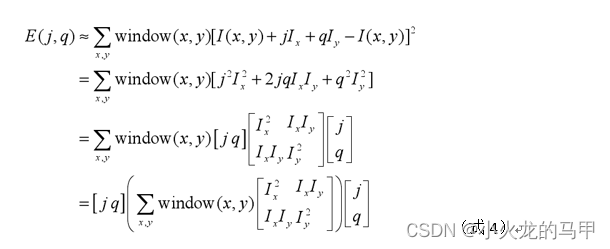其中，Ix、Iy分别为x、y方向上的偏导数
可将式（4）简写成：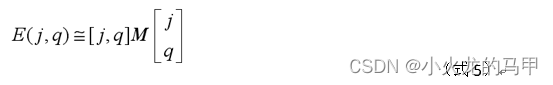其中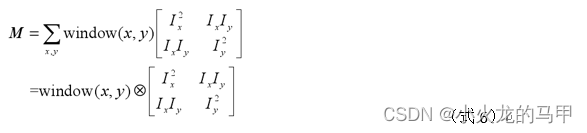矩阵M也可以进一步写成：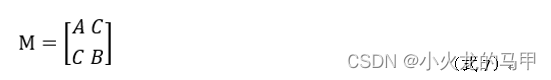其中，A、B、C分别是 I x 2 I_x^2 I y 2 I_y^2 I x y I_xy 在窗口w内的求和，即：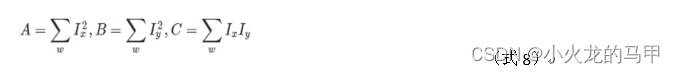上述就得到自相关函数的表达式。据文献4，这是一椭圆的矩阵表达形式（非标准椭圆），所以系数矩阵M的特征值与椭圆的半轴长短有关。而椭圆的半轴长短也影响到曲率，也即M的特征值会影响到自相关函数的变化快慢。如何影响？可看文献4的讲解（笔者数学不好，这里也是一知半解）。
根据文献4，对于一标准椭圆，其表达式为：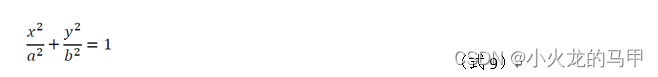矩阵形式为：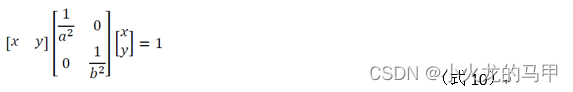则系数矩阵：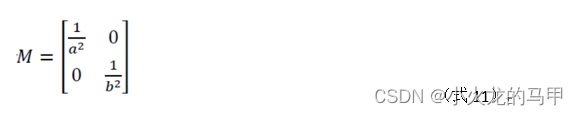则特征值与半轴的关系为：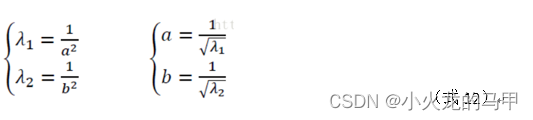结论是：特征值大，半轴短。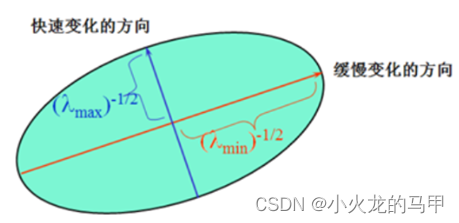便得到边界、平面、角点几种情况下，特征值的大小情况：
（1）边界。一个特征值大，另一个特征值小，λ1 >> λ2 或者 λ2 >> λ1。自相关函数值在某一个方向上大，在其他方向上小。
（2）平面。两个特征值都小，且近似相等；自相关函数数值在各个方向上都小。
（3）角点。两个特征值都很大且近似相等，自相关函数在所有方向都增大。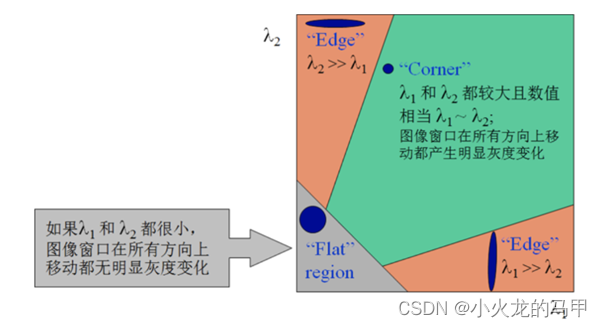实际中，Harris算法并不需要计算具体的特征值，而是根据角点响应值R来判断角点，R的定义为：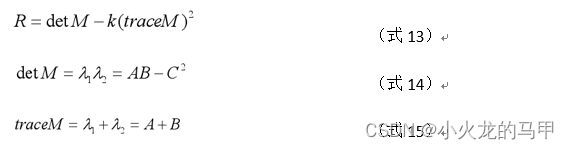其中，detM为矩阵M的行列式，traceM为矩阵M的迹，k为Harris算子参数，据文献3，其一般取值为[0.04，0.06]。据文献5，角点响应值判断角点的依据如下：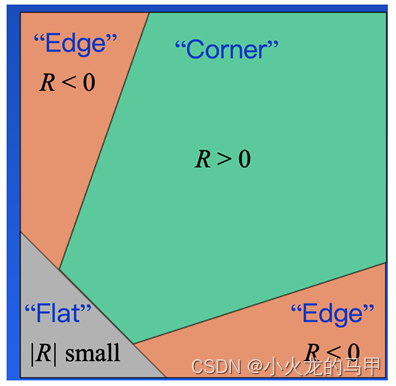即：
（1）当R为大数值的正数时是角点
（2）当R为大数值的负数时是边界
（3）当R为小数是认为是平坦区域
综上，Harris 算法分以下几个步骤：
（1）计算图像在水平方向和垂直方向的导数 I x Ix I y Iy 以及 I x 2 I_x^2 I y 2 I_y^2 I x y I_xy
（2）根据式（8）对上述元素进行平滑滤波，以得到系数 A、B、C，得到M。
（3）将求得的系数带入式（13）~式（15），来计算角点响应值 R。
（4）对于各响应值R，若大于设定好的阈值，则该R对应的坐标即为角点。

# 2、python源码实现

参阅文献6，基于python自行编写代码实现harris角点检测。几点需要注意的地方：
（1）通过sobel算子，快速实现图像x、y方向上导数的求取；
（2）opencv在实现harris算子时，窗口函数并非取的高斯函数，而是普通的boxFilter函数（即权值均为1，且求和后，不归一化）。
引用库：

#coding=utf8
import numpy as np
from PIL import Image
import matplotlib.pyplot as plt
import matplotlib.cm as cm
import math
import cv2
import os,sys
import scipy.ndimage
import time
import scipy


harris角点检测代码：

bash
def harris_corner_detect(img_src,block_size=2,aperture_size=3,k=0.04,borderType=cv2.BORDER_DEFAULT):
if img_src.dtype!=np.uint8:
raise ("input image shoud be uint8 type")
R_arr = np.zeros(img_src.shape,dtype=np.float32)#用来存储角点响应值
img = img_src.astype(np.float32)
scale = 1.0/( (aperture_size-1)*2*block_size*255 )#参考opencv实现源码，在sobel算子时乘以这个系数
#借助sobel算子，计算x、y方向上的偏导数
Ix = cv2.Sobel(img,-1,dx=1,dy=0,ksize=aperture_size,scale=scale,borderType=borderType)
Iy = cv2.Sobel(img,-1,dx=0,dy=1,ksize=aperture_size,scale=scale,borderType=borderType)
Ixx = Ix**2
Iyy = Iy**2
Ixy = Ix*Iy
#借助boxFilter函数，以block_size为窗口大小，对窗口内的数值求和，且不归一化
f_xx = cv2.boxFilter(Ixx,ddepth=-1,ksize=(block_size,block_size) ,anchor =(-1,-1),normalize=False, borderType=borderType)
f_yy = cv2.boxFilter(Iyy,ddepth=-1,ksize=(block_size,block_size),anchor =(-1,-1),normalize=False,borderType=borderType)
f_xy = cv2.boxFilter(Ixy, ddepth=-1,ksize=(block_size,block_size),anchor =(-1,-1),normalize=False,borderType=borderType)
# 也可以尝试手动求和
radius = int((block_size - 1) / 2)  # 考虑blocksize为偶数的情况，奇数时，前、后的数量一样；为偶数时，后比前多一个
N_post = block_size - N_pre - 1
row_s, col_s = N_pre, N_pre
row_e, col_e = img.shape - N_post, img.shape - N_post
#开始计算每一个坐标下的响应值
for r in range(row_s,row_e):
for c in range(col_s,col_e):
#手动对窗口内的数值求和
#sum_xx = Ixx[r-N_pre:r+N_post+1,c-N_pre:c+N_post+1].sum()
#sum_yy = Iyy[r-N_pre:r+N_post+1,c-N_pre:c+N_post+1].sum()
#sum_xy = Ixy[r-N_pre:r+N_post+1,c-N_pre:c+N_post+1].sum()
#或者直接使用boxFilter的结果
sum_xx = f_xx[r,c]
sum_yy = f_yy[r, c]
sum_xy = f_xy[r, c]
#根据行列式和迹求响应值
det = (sum_xx * sum_yy) - (sum_xy ** 2)
trace = sum_xx + sum_yy
res = det - k * (trace ** 2)
# 或者用如下方式求行列式和迹
#M = np.array([[sum_xx,sum_xy],[sum_xy,sum_yy]])
#res = np.linalg.det(M) - (k *  (np.trace(M))**2 )
R_arr[r,c] = res
return R_arr


主程序调用：
这里，响应值的门限选择为最大响应值得0.01倍。

if __name__ == '__main__':
#source code
res_arr = harris_corner_detect(img_src,block_size=2,aperture_size=3,k=0.04)
max_v = np.max(res_arr)
res_arr[res_arr<0.01*max_v]=0
img_show = img_src.copy()
if(len(img_show.shape)==2):
img_show = cv2.cvtColor(img_show,cv2.COLOR_GRAY2BGR)
img_show[res_arr!=0] = (255,0,0)
print(len(np.where(res_arr!=0)))
print(np.max(res_arr))
plt.figure()
plt.title("corners-raw")
plt.imshow(img_show, cmap=cm.gray)
plt.show()


检测结果如下：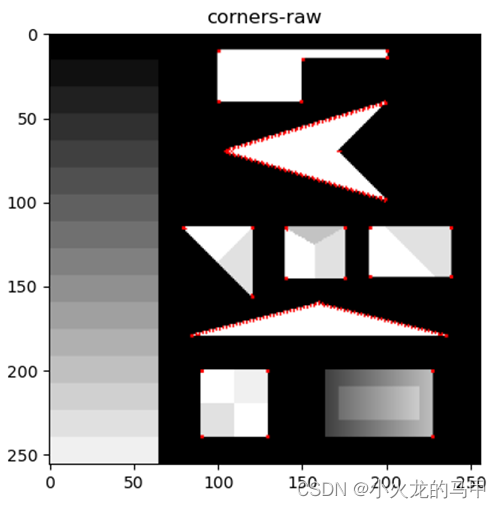# 3、基于opencv实现

Opencv自带harris算子，可以直接求取响应矩阵。这里使用的opencv版本为3.4.2.16
调用方式为：
dst = cv2.cornerHarris(img_src,blockSize= 2,ksize= 3,k= 0.04)
主程序调用：

if __name__ == '__main__':
#source code
res_arr = harris_corner_detect(img_src,block_size=2,aperture_size=3,k=0.04)
max_v = np.max(res_arr)
res_arr[res_arr<0.01*max_v]=0
img_show = img_src.copy()
if(len(img_show.shape)==2):
img_show = cv2.cvtColor(img_show,cv2.COLOR_GRAY2BGR)
img_show[res_arr!=0] = (255,0,0)
print(len(np.where(res_arr!=0)))
print(np.max(res_arr))
plt.figure()
plt.title("corners-raw")
plt.imshow(img_show, cmap=cm.gray)
#opencv库函数调用
dst = cv2.cornerHarris(img_src,blockSize= 2,ksize= 3,k= 0.04) #blockSize为窗口大小，ksize为sobel算子的核大小，k为harris算子参数
img_show2 = img_src.copy()
if (len(img_show2.shape) == 2):
img_show2 = cv2.cvtColor(img_show2, cv2.COLOR_GRAY2BGR)
dst2 = dst.copy()
dst2[dst<= 0.01* dst.max()]=0
img_show2[dst2!=0] = (255, 0, 0)
print(len(np.where(dst2 != 0)))
print(np.max(dst2))
plt.figure()
plt.title("opencv")
plt.imshow(img_show2, cmap=cm.gray)
plt.show()


opencv库函数检测结果如下：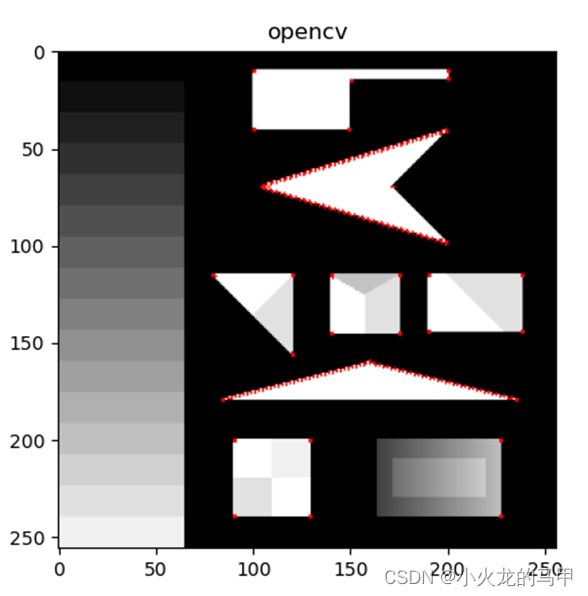将自行编写的harris角点检测代码运行结果与opencv运行结果相对比，二者一致（仅仅比较了数量、最大值）。

# 4、非极大值抑制

可根据需要，执行非极大值抑制。

非极大值抑制代码：

def corner_nms(corner,kernal=3):
out = corner.copy()
row_s = int(kernal/2)
row_e = out.shape - int(kernal/2)
col_s,col_e = int(kernal/2),out.shape - int(kernal/2)
for r in range(row_s,row_e):
for c in range(col_s,col_e):
if corner[r,c]==0: #不是可能的角点
continue
zone = corner[r-int(kernal/2):r+int(kernal/2)+1,c-int(kernal/2):c+int(kernal/2)+1]
index = corner[r,c]<zone
(x,y) = np.where(index==True)
if len(x)>0 : #说明corner[r,c]不是最大，直接归零将其抑制
out[r,c] = 0
return out


在上文基础上，调用nms：

	#nms
score_nms = corner_nms(res_arr)
img_show3 = cv2.cvtColor(img_src,cv2.COLOR_GRAY2BGR)
img_show3[score_nms != 0] = (255, 0, 0)
plt.figure()
plt.title("corners-nms")
plt.imshow(img_show3, cmap=cm.gray)

plt.show()


经过nms抑制后的结果：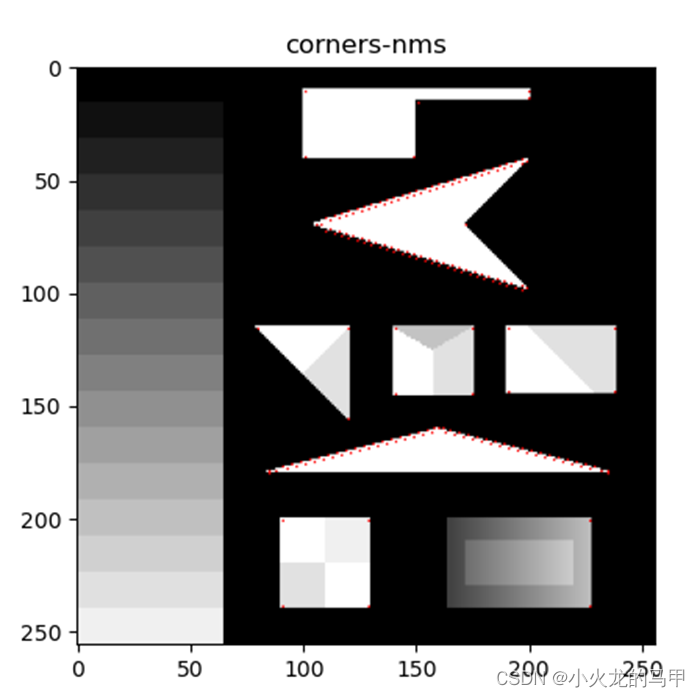# 5、python源码下载

Python程序源码下载地址

展开全文opencv python 计算机视觉
• 算法流程：将图像转换为灰度图像利用Sobel滤波器求出 海森矩阵 (Hessian matrix) :将高斯滤波器分别作用于Ix²、Iy²、IxIy计算每个像素的 R= det(H) - k(trace...=max(R) * th 的像素即为角点。th常取0.1。Harri...

算法流程：

将图像转换为灰度图像

利用Sobel滤波器求出 海森矩阵 (Hessian matrix) :将高斯滤波器分别作用于Ix²、Iy²、IxIy

计算每个像素的 R= det(H) - k(trace(H))²。det(H)表示矩阵H的行列式，trace表示矩阵H的迹。通常k的取值范围为[0.04,0.16]。

满足 R>=max(R) * th 的像素点即为角点。th常取0.1。

Harris算法实现：

import cv2 as cv

import numpy as np

import matplotlib.pyplot as plt

# Harris corner detection

def Harris_corner(img):

## Grayscale

def BGR2GRAY(img):

gray = 0.2126 * img[..., 2] + 0.7152 * img[..., 1] + 0.0722 * img[..., 0]

gray = gray.astype(np.uint8)

return gray

## Sobel

def Sobel_filtering(gray):

# get shape

H, W = gray.shape

# sobel kernel

sobely = np.array(((1, 2, 1),

(0, 0, 0),

(-1, -2, -1)), dtype=np.float32)

sobelx = np.array(((1, 0, -1),

(2, 0, -2),

(1, 0, -1)), dtype=np.float32)

tmp = np.pad(gray, (1, 1), 'edge')

# prepare

Ix = np.zeros_like(gray, dtype=np.float32)

Iy = np.zeros_like(gray, dtype=np.float32)

# get differential

for y in range(H):

for x in range(W):

Ix[y, x] = np.mean(tmp[y : y + 3, x : x + 3] * sobelx)

Iy[y, x] = np.mean(tmp[y : y + 3, x : x + 3] * sobely)

Ix2 = Ix ** 2

Iy2 = Iy ** 2

Ixy = Ix * Iy

return Ix2, Iy2, Ixy

# gaussian filtering

def gaussian_filtering(I, K_size=3, sigma=3):

# get shape

H, W = I.shape

## gaussian

I_t = np.pad(I, (K_size // 2, K_size // 2), 'edge')

# gaussian kernel

K = np.zeros((K_size, K_size), dtype=np.float)

for x in range(K_size):

for y in range(K_size):

_x = x - K_size // 2

_y = y - K_size // 2

K[y, x] = np.exp( -(_x ** 2 + _y ** 2) / (2 * (sigma ** 2)))

K /= (sigma * np.sqrt(2 * np.pi))

K /= K.sum()

# filtering

for y in range(H):

for x in range(W):

I[y,x] = np.sum(I_t[y : y + K_size, x : x + K_size] * K)

return I

# corner detect

def corner_detect(gray, Ix2, Iy2, Ixy, k=0.04, th=0.1):

# prepare output image

out = np.array((gray, gray, gray))

out = np.transpose(out, (1,2,0))

# get R

R = (Ix2 * Iy2 - Ixy ** 2) - k * ((Ix2 + Iy2) ** 2)

# detect corner

out[R >= np.max(R) * th] = [255, 0, 0]

out = out.astype(np.uint8)

return out

# 1. grayscale

gray = BGR2GRAY(img)

# 2. get difference image

Ix2, Iy2, Ixy = Sobel_filtering(gray)

# 3. gaussian filtering

Ix2 = gaussian_filtering(Ix2, K_size=3, sigma=3)

Iy2 = gaussian_filtering(Iy2, K_size=3, sigma=3)

Ixy = gaussian_filtering(Ixy, K_size=3, sigma=3)

# 4. corner detect

out = corner_detect(gray, Ix2, Iy2, Ixy)

return out

# Harris corner detection

out = Harris_corner(img)

cv.imwrite("out.jpg", out)

cv.imshow("result", out)

cv.waitKey(0)

cv.destroyAllWindows()

实验结果：

原图：Harris角点检测算法检测结果：点个赞再走呗！

展开全文• Harris角点检测 概念 基于特征的图像配准方法是图像配准中最常见的方法之一。它不是直接利用图像像素值，二十通过像素值导出的符号特征（如特征、特征线、特征区域）来实现图像配准，因此可以克服利用灰度信息进行...

# Harris角点检测

## 概念

基于特征的图像配准方法是图像配准中最常见的方法之一。它不是直接利用图像像素值，二十通过像素值导出的符号特征（如特征点、特征线、特征区域）来实现图像配准，因此可以克服利用灰度信息进行图像配准的缺点，主要体现在以下三个方面：
（1）利用特征点而不是图像灰度信息，大大减少了在匹配过程中的计算量；
（2）特征点的匹配度量值相对位置变化比较敏感，可以提高匹配的精度；
（3）特征点的提取过程可以减少噪声的影响，对灰度

### 角点

一类重要的点特征：角点（corner points），其定义主要有以下：

（1）局部窗口沿各方向移动，灰度均产生明显变化的点
（2）图像局部曲率突变的点

我们可以直观的概括下角点所具有的特征：

轮廓之间的交点；

对于同一场景，即使视角发生变化，通常具备稳定性质的特征；

该点附近区域的像素点无论在梯度方向上还是其梯度幅值上有着较大变化；

### 角点检测

角点检测大致可分为三类：基于灰度图的角点检测、基于二值化图像的角点检测和基于轮廓曲线的角点检测。Harris属于基于灰度图的角点检测。

## 原理

### 算法的基本思想

算法基本思想是使用一个固定窗口在图像上进行任意方向上的滑动，比较滑动前与滑动后两种情况，窗口中的像素灰度变化程度，如果存在任意方向上的滑动，都有着较大灰度变化，那么我们可以认为该窗口中存在角点。

Harris角点检测可分为三步：梯度计算、响应值计算、角点提取。

#### 梯度计算

对图像中的任意一像素点I（x,y），进行自相关平移w(x+∆x、y+∆y)得到自相关函数：

c(x,y,∆x,∆y) = ∑wh(x,y)(I(x,y)-I(x+∆x,y+∆y))2

其中 ∑w表示窗口内的点，h(x,y)表示加权函数，加权函数可根据自己需要进行修改（通过修改源代码）。

由泰勒可得：

I(x+∆x,y+∆y) = I(x,y)+∆xIx(x,y)+∆yIy(x,y)+p ≈I(x,y)+∆xIx(x,y)+∆yIy(x,y)

        代入自相关函数可得（加权函数暂时忽略）：


c(x,y,∆x,∆y) = ∑w(I(x,y)-I(x+∆x,y+∆y))2 ≈ ∑w((∆xIx(x,y))2+2∆x∆yIx(x,y)Iy(x,y)+(∆yIy(x,y))2)

将上公式用图表示如下：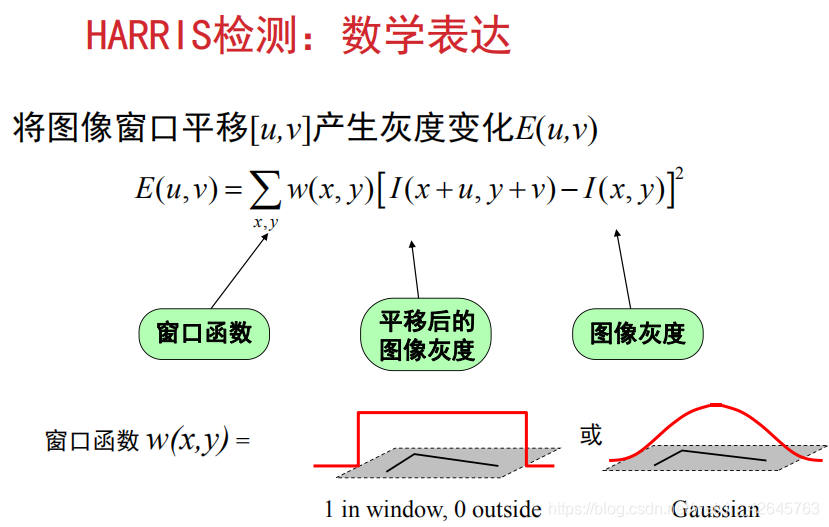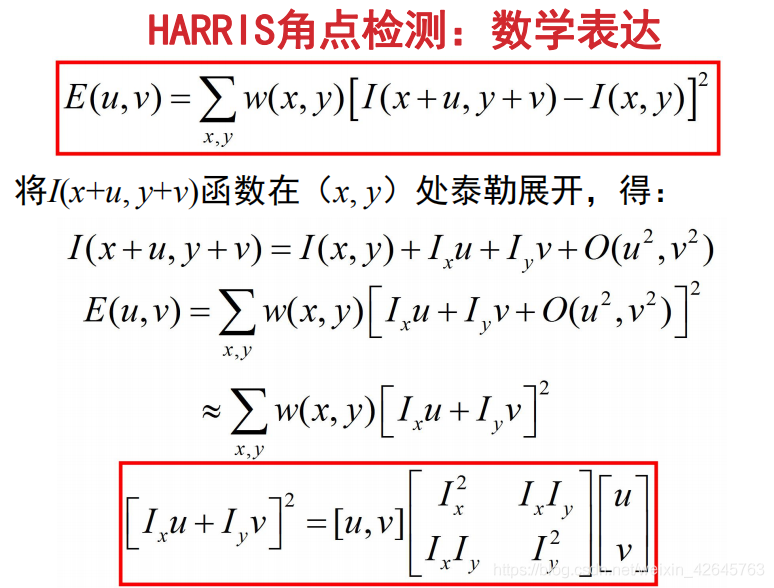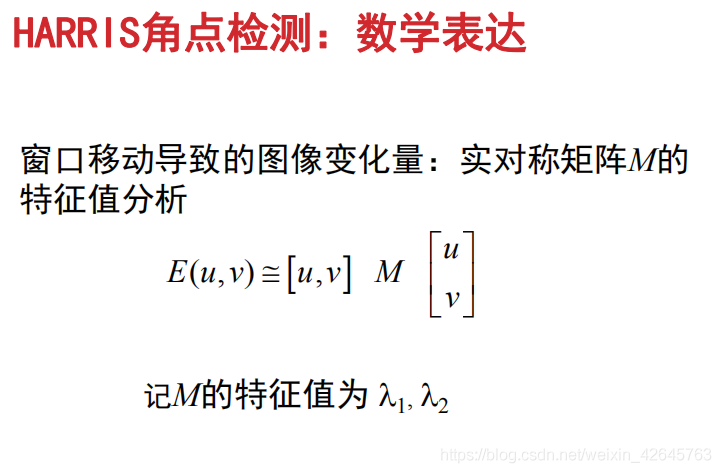其中，u和v分别表示∆x和∆y，w(x,y)表示加权函数。Harris算法是通过判断像素值是否在多个方向上有明显变化可转换为为是否在x和y方向上像素值均有明显变化，再转换为Ix或Iy的变化，再转换为M矩阵的特征值λ1,λ2的变化，如下图：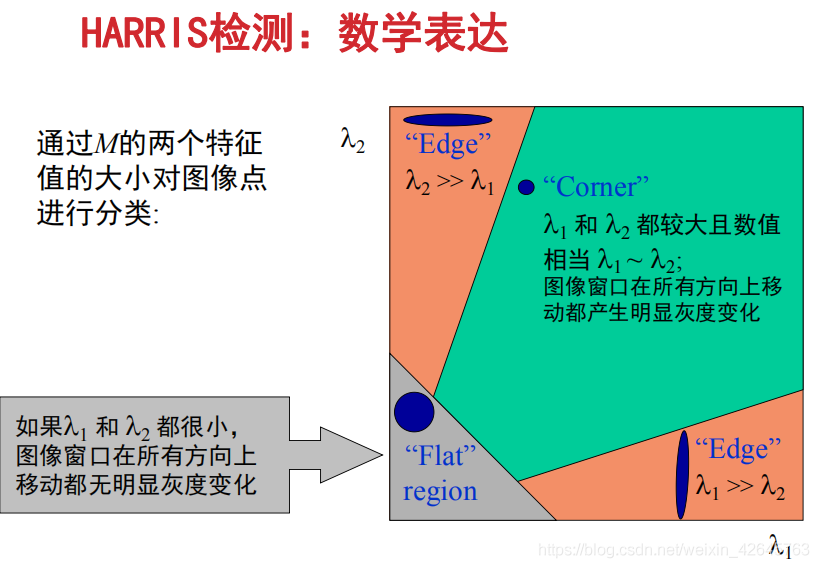#### 响应值计算

上面计算不易于通过编程实现，Harris通过定义角点响应函数R的方式，用于表示一个角点的Harris响应值，通常用下面表达式度量角点响应：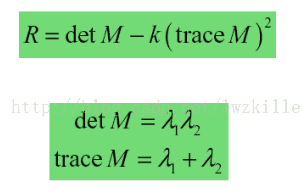trace表示为矩阵的迹，det为矩阵的行列式（矩阵的迹：主对角线上的值相加即所有特征值的和），k是常量，一般取值为0.04~0.06，这个参数仅仅是这个函数的一个系数，它的存在只是调节函数的形状而已。
R = λ1λ2-k(λ1+λ2)2

#### 角点提取

通过上面计算出一角点的Harris响应值大小，后通过设定的阈值t，进行判断该点是否为角点（大于t为角点，小于t则不为角点）

### Harris角点检测流程

（1）通过高斯函数的导数对原始图像进行卷积计算；图像在水平方向和垂直方向的导数Ix和Iy；
（2）计算对应这些梯度外积即（Ix2 、Iy2、IxIy）三个图像。
（3）使用高斯函数对以上图像进行卷积滤波；
（4）使用前面的公式计算角点响应函数R值；
（5）对计算到的角点图像进行局部极大值抑制。

## 实现

### 实验要求

（1）需要拍摄纹理平坦、垂直或水平边缘多（如建筑物）、纹理角点丰富的三种场景
（2）每种场景，至少需要有 正面、侧面、旋转、尺度（远近）变化，最好能再加光照变化
（3）每种场景实验结果结束后，做实验结果的分析，分析内容需涉及到原理与实验结果的对应关系

### 代码

# -*- coding: utf-8 -*-
from pylab import *
from PIL import Image
from PCV.localdescriptors import harris

"""
Example of detecting Harris corner points (Figure 2-1 in the book).
"""

# 读入图像
im = array(Image.open('../data/empire.jpg').convert('L'))

# 检测harris角点
harrisim = harris.compute_harris_response(im)

# Harris响应函数
harrisim1 = 255 - harrisim

figure()
gray()

#画出Harris响应图
subplot(141)
imshow(harrisim1)
print harrisim1.shape
axis('off')
axis('equal')

threshold = [0.01, 0.05, 0.1]
for i, thres in enumerate(threshold):
filtered_coords = harris.get_harris_points(harrisim, 6, thres)
subplot(1, 4, i+2)
imshow(im)
print im.shape
plot([p for p in filtered_coords], [p for p in filtered_coords], '*')
axis('off')

#原书采用的PCV中PCV harris模块
#harris.plot_harris_points(im, filtered_coords)

# plot only 200 strongest
# harris.plot_harris_points(im, filtered_coords[:200])

show()

`

### 结果

#### 1.边缘丰富区域

##### 1.1正面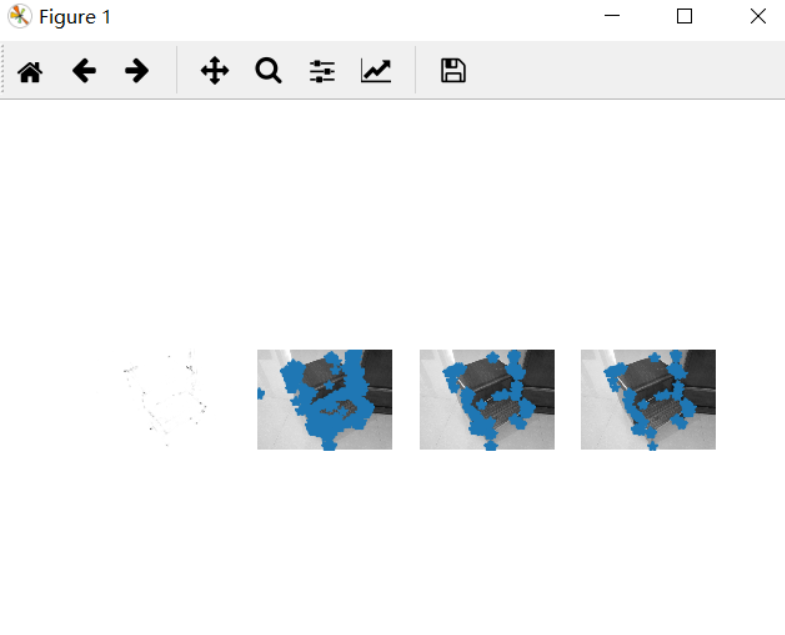##### 1.2侧面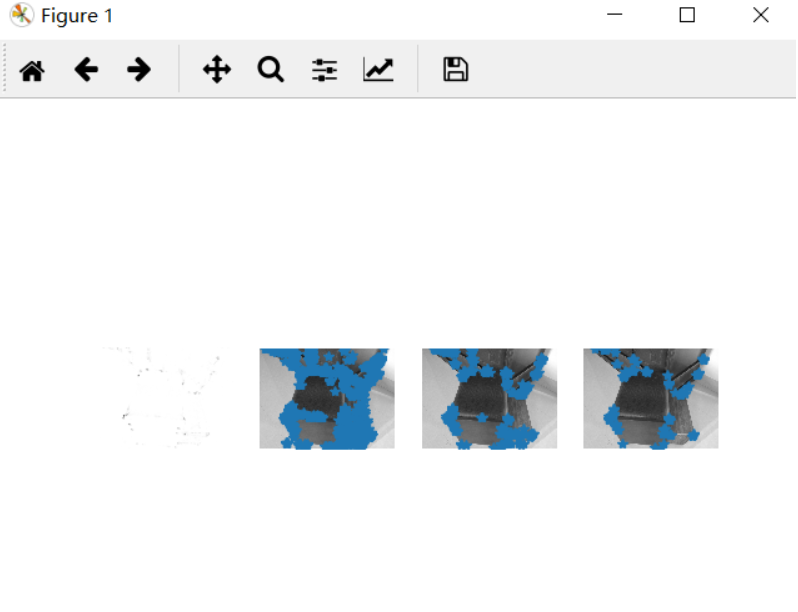##### 1.3旋转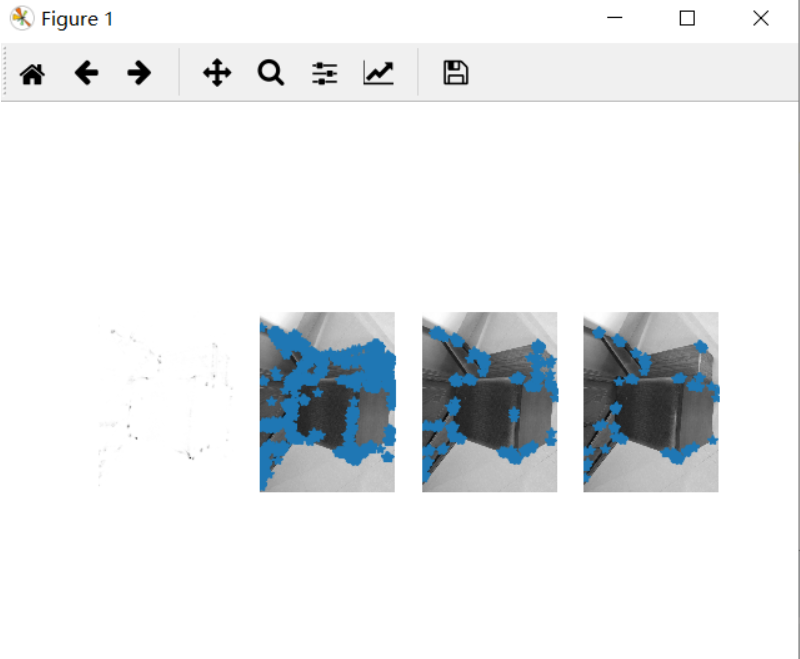##### 1.4远近对比（近）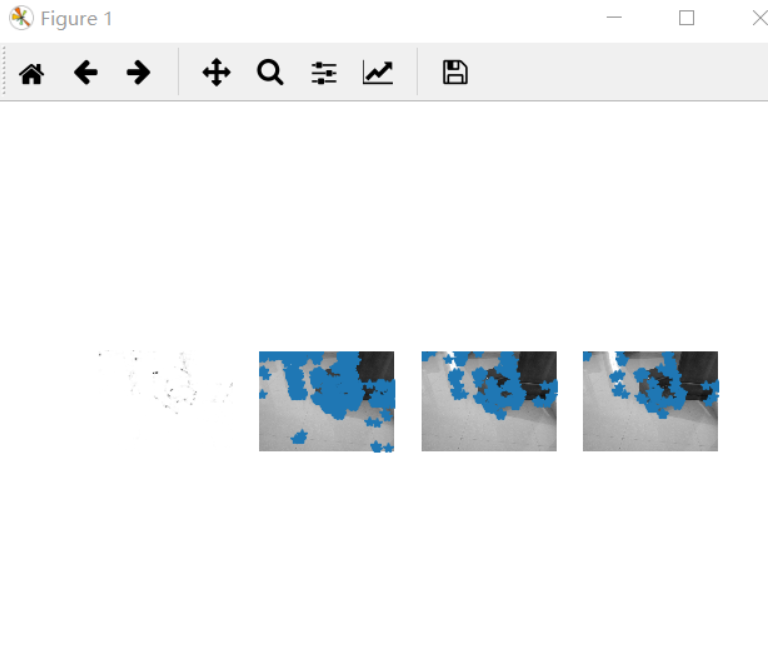##### 1.5光暗对比（暗）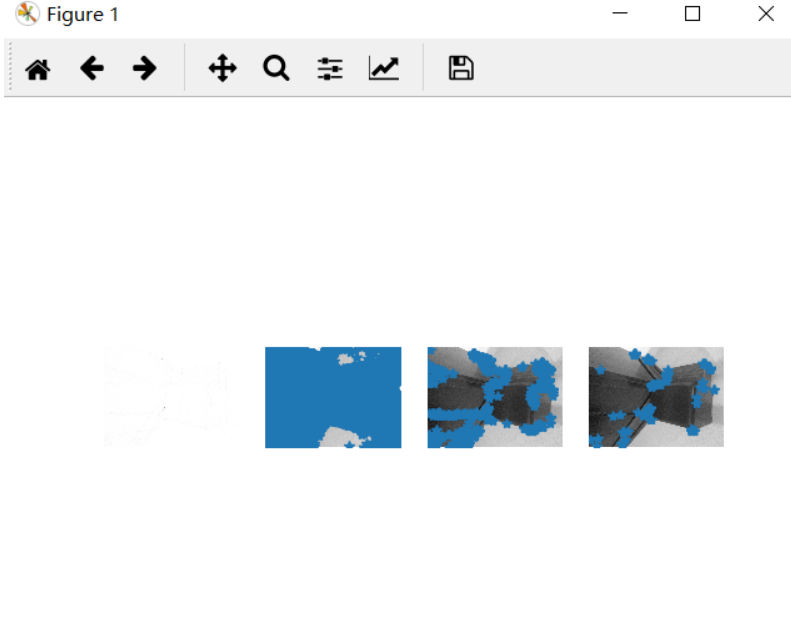分析：由该组实验可知，边缘丰富区域多的图片角点较多。明亮暗程度会影响角点的寻找，较亮的事物更精确。

#### 2.纹理角点丰富区域

##### 2.1正面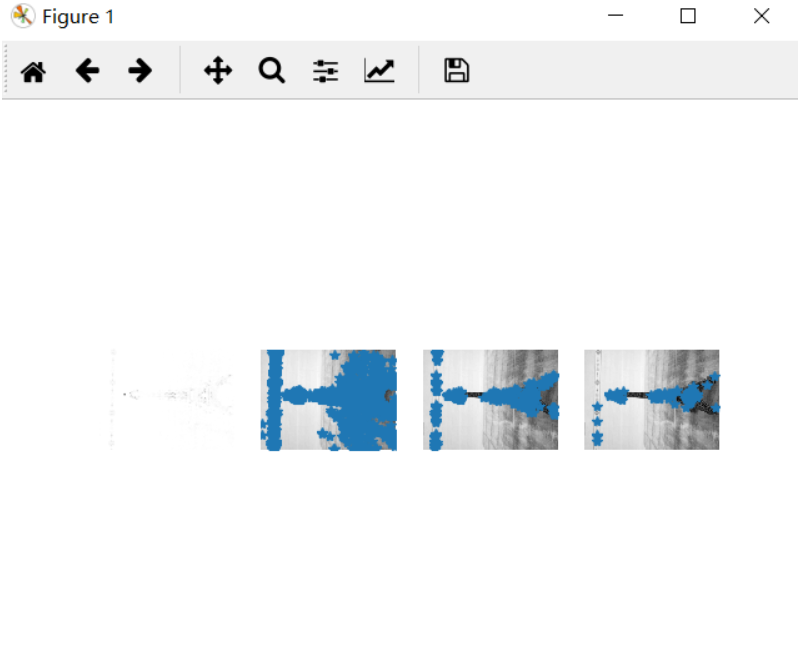##### 2.2侧面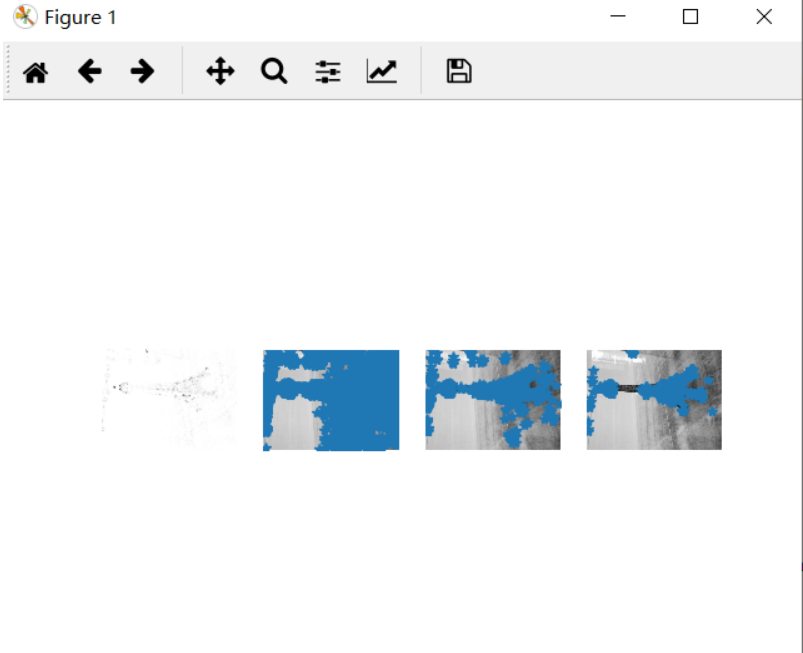##### 2.3旋转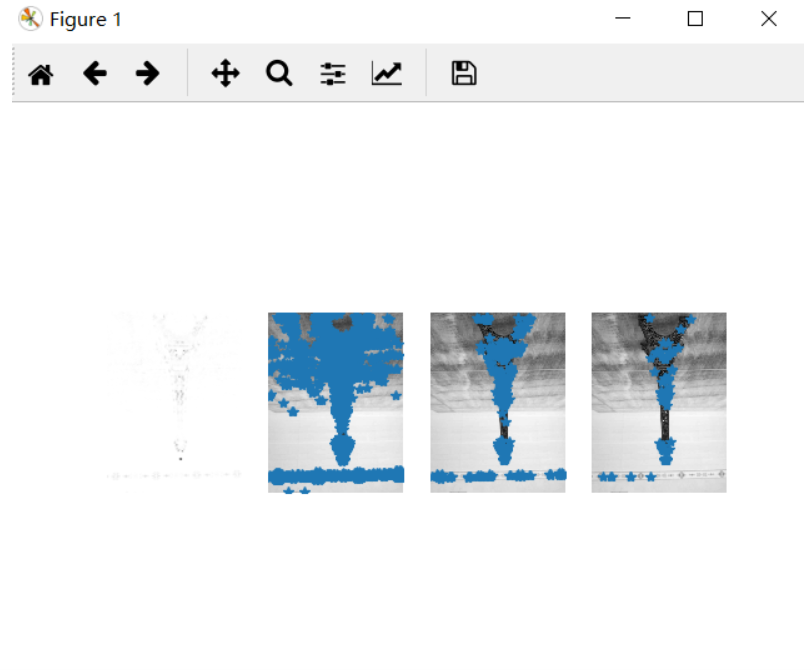##### 2.4远近对比（远）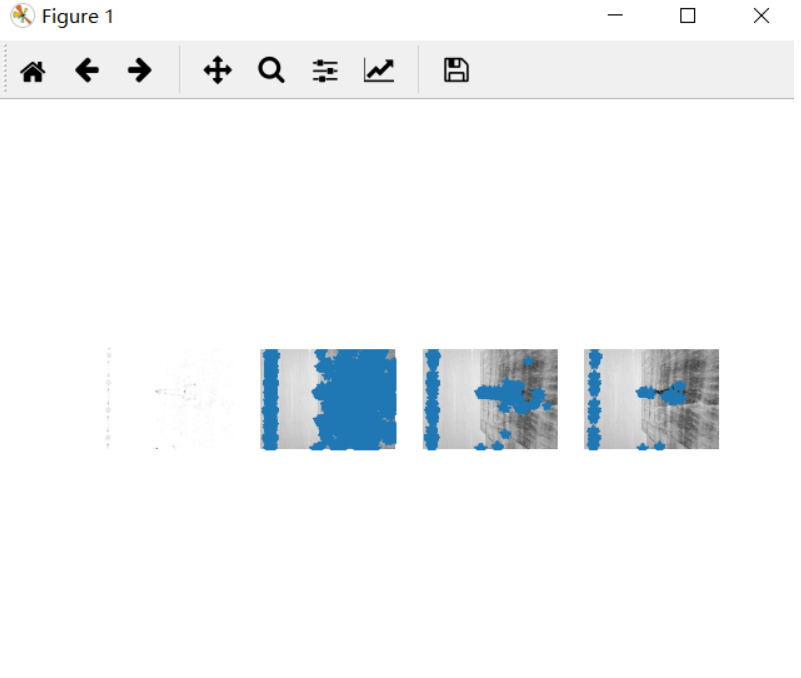##### 2.5光暗对比（暗）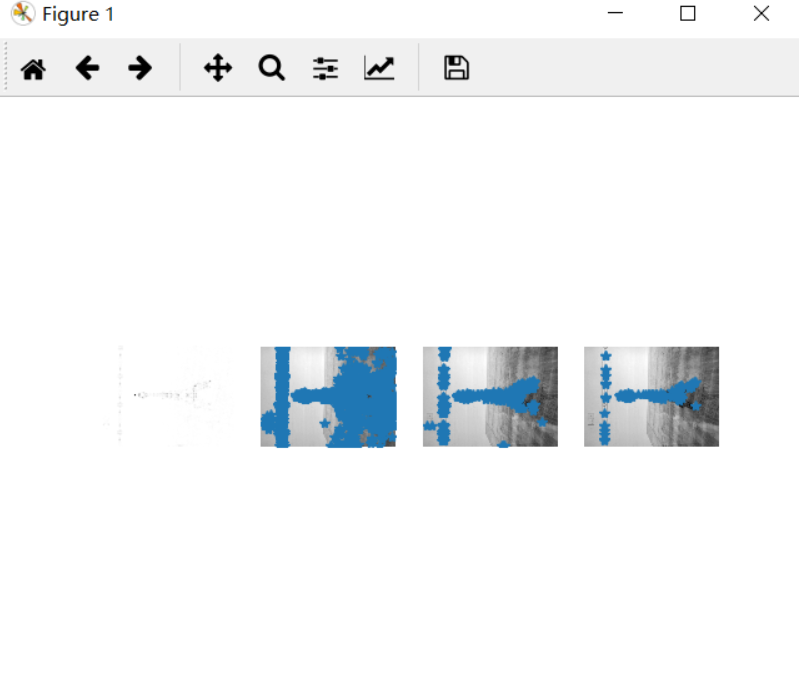由该组实验可知，纹理丰富的图片角点更甚于边缘丰富图片，远近程度会影响纹理丰富的图片寻找角点，亮暗程度影响纹理丰富的图片寻找角点的程度更高，光亮的图片更有利于寻找角点。

#### 3.纹理平坦区域

##### 3.1正面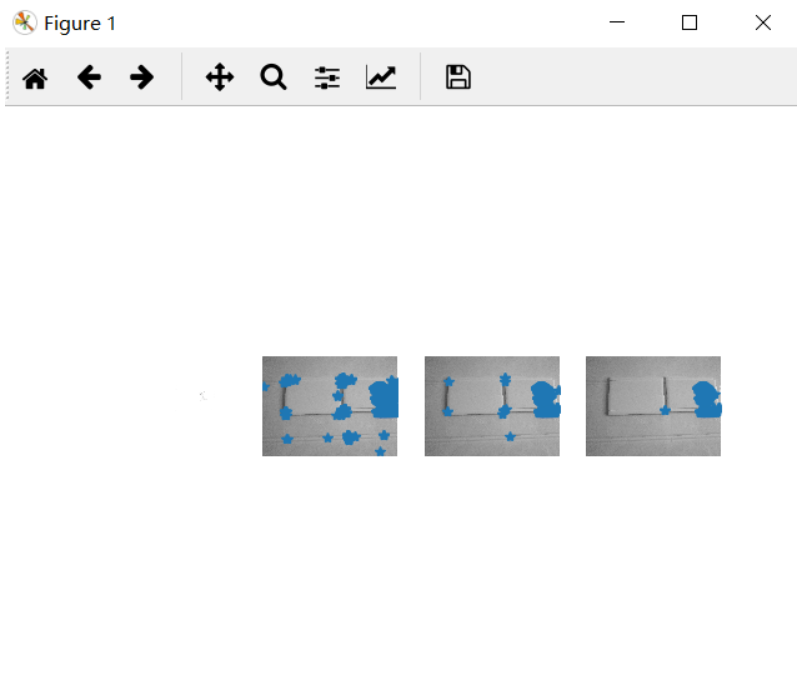##### 3.2侧面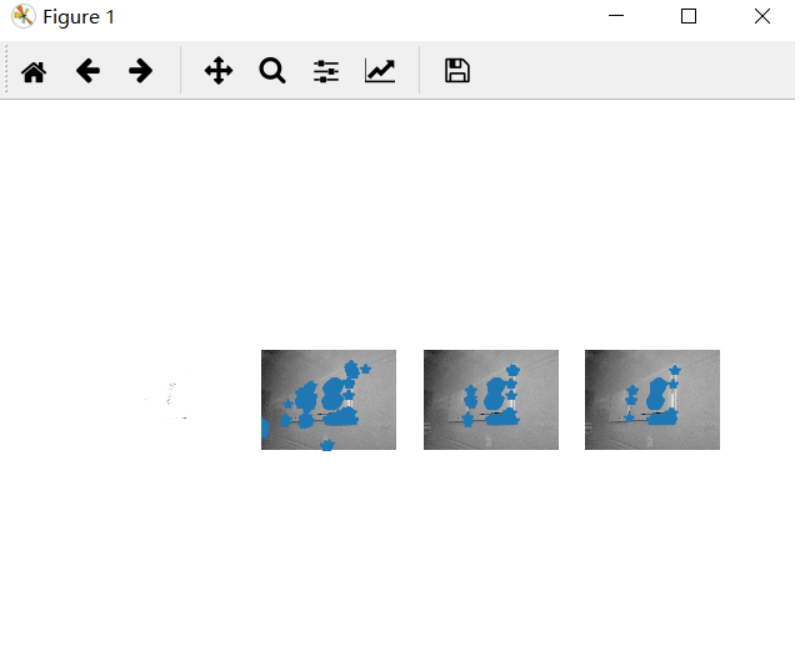##### 3.3旋转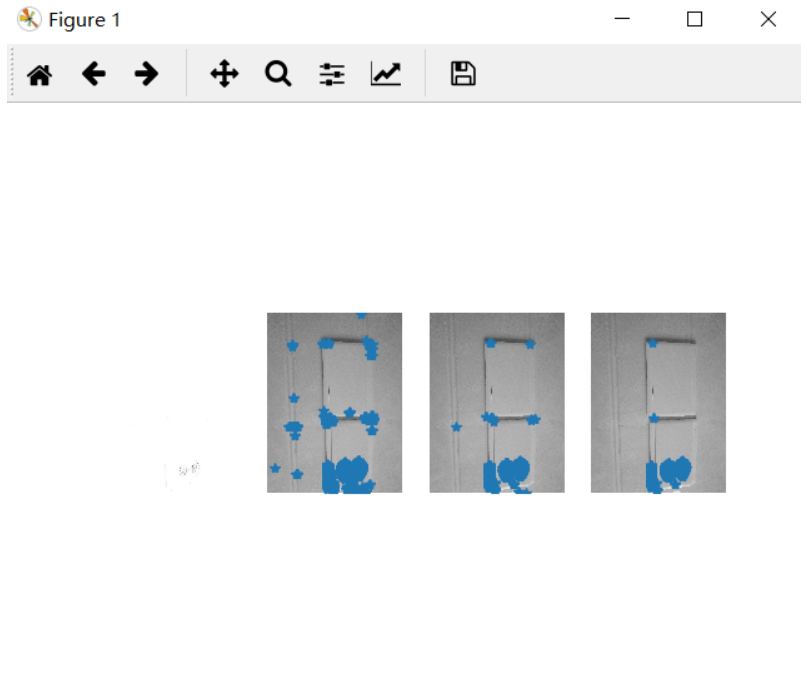##### 3.4远近对比（远）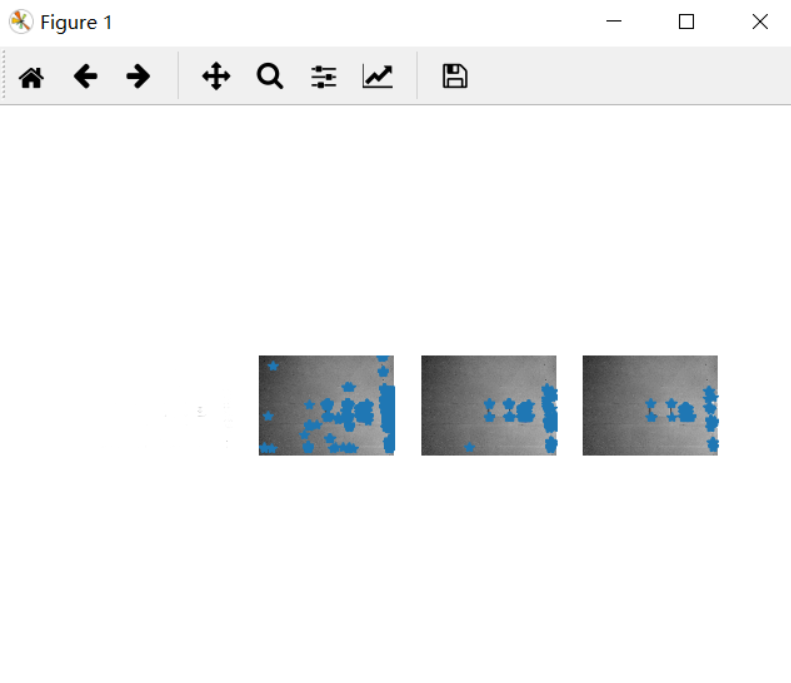##### 3.5光暗对比（暗）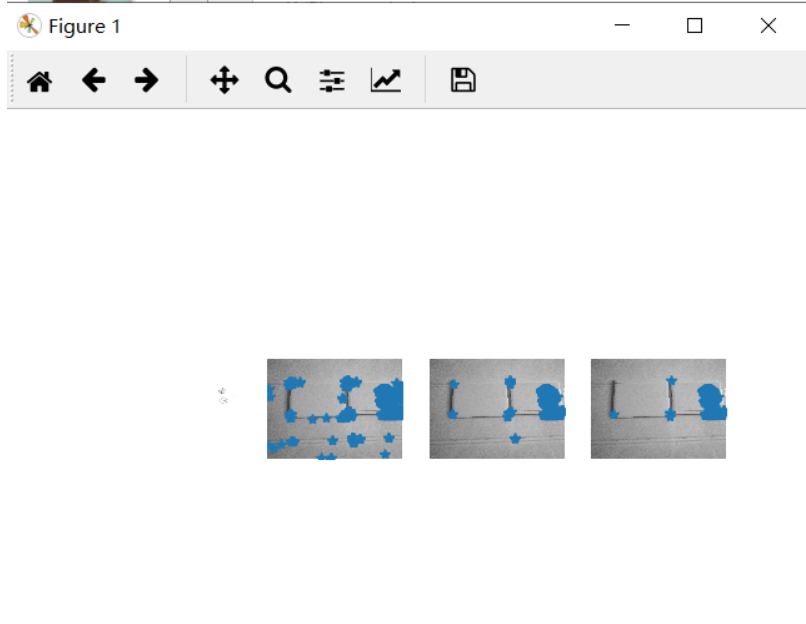由该组实验可知，纹理平坦的图片角点较少。对于纹理平坦的图片来讲，寻找角点，图片的角度，远近程度，明暗程度都不大影响纹理平坦的图片寻找角点。

# 借鉴

展开全文•python opencv 计算机视觉
• 算法流程：将图像转换为灰度图像利用Sobel滤波器求出 海森矩阵 (Hessian matrix) :将高斯滤波器分别作用于Ix²、Iy²、IxIy计算每个像素的 R= det(H) - k(trace...=max(R) * th 的像素即为角点。th常取0.1。Harri...
• 在学习时主要参考了1.http://blog.csdn.net/xiaowei_cqu/article/details/7805206和opencv-python官方的关于harris的文档...harris/py_f特征点检测 角点检测 opencv
• Harris 角点检测 特征匹配 C++基于Harris的特征检测与匹配
• Harris角点检测(Python实现) 1. 简介 &nbsp;角是图像很重要的特征,对图像图形的理解和分析有很重要的作用。角在保留图像图形重要特征的同时,可以有效地减少信息的数据量,使其信息的含量很高,有效地提高...Harris角点检测 Python OpenCV
• 克里斯·哈里斯（Chris Harris）和迈克·史蒂芬斯（Mike Stephens）在1988年的论文《组合式拐角和边缘检测器》中做了一次尝试找到这些拐角的尝试，所以现在将其称为哈里斯拐角检测器。 函数：cv2.cornerHarris（），...
• 一实验原理1.1角点1.2角点检测算法的好处1.3Harris算法1.3.1Harris算法1.3.2数学表达二、代码实现及结果截图及实验分析三、实验总结1.1角点局部窗口沿各方向移动，均产生明显变化的，图像局部曲率突变的，是在...
• 算法流程： 1、将图像转换为灰度图像； 2、利用Sobel滤波器求出 海森矩阵 (Hessian matrix) : 3、将高斯滤波器分别作用于Ix²、Iy²、IxIy；...4、计算每个像素的 R= ...=max(R) * th 的像素即为角点。th常取0...python opencv 计算机视觉 目标检测
• 是一种基于图像灰度的方法通过计算的曲率和梯度来检测角点。 基本原理 如果在各个方向上移动窗口，窗口中的灰度值都会发生较大变化，那么认定在窗口遇到了角点； 如果在一个方向发生变化，另一个方向不变，就可能...
• 文章目录第二章 局部图像描绘子2.1 Harris角点检测器描述子信息 第二章 局部图像描绘子 2.1 Harris角点检测器 1、角定义： a.局部窗口沿各方向移动，均产生明显变化的 b.图像局部曲率突变的 2、xxx的...计算机视觉 opencv python
• 目录一、背景介绍1.1 什么是特征1.2 为什么做特征检测1.3 特征检测的方法二、Harris角点检测原理三、基于python-opencv实现Harris角点检测 一、背景介绍 1.1 什么是特征 在数据挖掘、计算机视觉等领域经常会提起...计算机视觉 python 人工智能
• OpenCV——harris角点检测算法的C++与python代码实现opencv python
• 重磅干货，第一时间送达 特征又叫兴趣或者角。常被用于目标匹配，目标跟踪，三维重建等应用中。特征主要指图像中的明显，如突出的角、边缘端点、极值等等。...常用的有Harris角点检测； FAST特征检测...python 计算机视觉 机器学习 人工智能
• Shi-tomasi角点检测python实现及基于opencv实现（角点检测、非极大值抑制）
• 角点就是极值，即在某方面属性特别突出的，是在某些属性上强度最大或者最小的孤立、线段的终点。而对于图像而言，如图所示红点部分，即为图像的角点，其是物体轮廓线的连接。 因此，在角点时，窗口向任意......